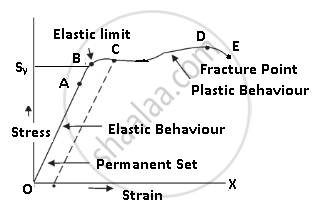# Stress-strain Curve

## Notes

Stress-Strain Curve:It is a curve between stress and strain.

• A graph is plotted between the stress (which is equal in magnitude to the applied force per unit area) and the strain produced.

• The graph helps us to understand how a given material deforms with increasing loads.

• The curve between O and A is a straight line. This means stress is directly ∝ to strain. In this region Hooke’s Law is applicable.

• In this region, the material behaves like an elastic body.

• In the region from A to B, stress, and strain are not directly proportional. But still, the material returns to its original dimension after the force is removed. They exhibit elastic properties.

• The point B in the curve is known as yield point (also known as elastic limit) which means till this point the material will be elastic in behavior and the stress corresponding to point B is known as yield strength (Sy) of the material.

• The region between O and B is called an Elastic region.

• From point B to point D, we can see that strain increases rapidly even for a small change in stress.

• Even if we remove the force the material does not come back to its original position. At this point, stress is zero but the strain is not zero as the body has changed its shape.

• The material has undergone plastic deformation.

• The material is said to be a permanent set.

• The point D on the graph is known as the ultimate tensile strength (Su) of the material.

• From D to E we can see that stress decreases even if strain increases.

• Finally at point E fracture occurs. This means the body breaks.

If you would like to contribute notes or other learning material, please submit them using the button below.